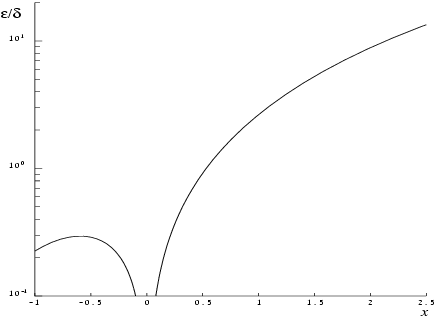NAG Library Function Document

1Purpose

nag_erfc (s15adc) returns the value of the complementary error function, $\mathrm{erfc}\left(x\right)$.

2Specification

 #include #include
 double nag_erfc (double x)

3Description

nag_erfc (s15adc) calculates an approximate value for the complement of the error function
 $erfcx=2π∫x∞e-t2dt=1-erfx.$
Let $\stackrel{^}{x}$ be the root of the equation $\mathrm{erfc}\left(x\right)-\mathrm{erf}\left(x\right)=0$ (then $\stackrel{^}{x}\approx 0.46875$). For $\left|x\right|\le \stackrel{^}{x}$ the value of $\mathrm{erfc}\left(x\right)$ is based on the following rational Chebyshev expansion for $\mathrm{erf}\left(x\right)$:
 $erfx≈xRℓ,mx2,$
where ${R}_{\ell ,m}$ denotes a rational function of degree $\ell$ in the numerator and $m$ in the denominator.
For $\left|x\right|>\stackrel{^}{x}$ the value of $\mathrm{erfc}\left(x\right)$ is based on a rational Chebyshev expansion for $\mathrm{erfc}\left(x\right)$: for $\stackrel{^}{x}<\left|x\right|\le 4$ the value is based on the expansion
 $erfcx≈ex2Rℓ,mx;$
and for $\left|x\right|>4$ it is based on the expansion
 $erfcx≈ex2x1π+1x2Rℓ,m1/x2.$
For each expansion, the specific values of $\ell$ and $m$ are selected to be minimal such that the maximum relative error in the expansion is of the order ${10}^{-d}$, where $d$ is the maximum number of decimal digits that can be accurately represented for the particular implementation (see nag_decimal_digits (X02BEC)).
For $\left|x\right|\ge {x}_{\mathrm{hi}}$ there is a danger of setting underflow in $\mathrm{erfc}\left(x\right)$ (the value of ${x}_{\mathrm{hi}}$ is given in the Users' Note for your implementation). For $x\ge {x}_{\mathrm{hi}}$, nag_erfc (s15adc) returns $\mathrm{erfc}\left(x\right)=0$; for $x\le -{x}_{\mathrm{hi}}$ it returns $\mathrm{erfc}\left(x\right)=2$.
Abramowitz M and Stegun I A (1972) Handbook of Mathematical Functions (3rd Edition) Dover Publications
Cody W J (1969) Rational Chebyshev approximations for the error function Math.Comp. 23 631–637

5Arguments

1:    $\mathbf{x}$doubleInput
On entry: the argument $x$ of the function.

None.

7Accuracy

If $\delta$ and $\epsilon$ are relative errors in the argument and result, respectively, then in principle
 $ε≃ 2x e -x2 πerfcx δ .$
That is, the relative error in the argument, $x$, is amplified by a factor $\frac{2x{e}^{-{x}^{2}}}{\sqrt{\pi }\mathrm{erfc}\left(x\right)}$ in the result.
The behaviour of this factor is shown in Figure 1.Figure 1
It should be noted that near $x=0$ this factor behaves as $\frac{2x}{\sqrt{\pi }}$ and hence the accuracy is largely determined by the machine precision. Also for large negative $x$, where the factor is $\text{}\sim \frac{x{e}^{-{x}^{2}}}{\sqrt{\pi }}$, accuracy is mainly limited by machine precision. However, for large positive $x$, the factor becomes $\text{}\sim 2{x}^{2}$ and to an extent relative accuracy is necessarily lost. The absolute accuracy $E$ is given by
 $E≃2xe-x2πδ$
so absolute accuracy is guaranteed for all $x$.

None.

10Example

This example reads values of the argument $x$ from a file, evaluates the function at each value of $x$ and prints the results.